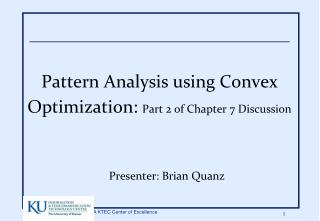DownloadDownload PresentationPattern Analysis using Convex Optimization: Part 2 of Chapter 7 Discussion

# Pattern Analysis using Convex Optimization: Part 2 of Chapter 7 Discussion

Download Presentation## Pattern Analysis using Convex Optimization: Part 2 of Chapter 7 Discussion

- - - - - - - - - - - - - - - - - - - - - - - - - - - E N D - - - - - - - - - - - - - - - - - - - - - - - - - - -
##### Presentation Transcript

1. Pattern Analysis using Convex Optimization: Part 2 of Chapter 7 Discussion Presenter: Brian Quanz

2. About today’s discussion… • Last time: discussed convex opt. • Today: Will apply what we learned to 4 pattern analysis problems given in book: • (1) Smallest enclosing hypersphere (one-class SVM) • (2) SVM classification • (3) Support vector regression (SVR) • (4) On-line classification and regression

3. About today’s discussion… • This time for the most part: • Describe problems • Derive solutions ourselves on the board! • Apply convex opt. knowledge to solve • Mostly board work today

4. Recall: KKT Conditions • What we will use: • Key to remember ch. 7: • Complementary slackness -> sparse dual rep. • Convexity -> efficient global solution

5. Novelty Detection: Hypersphere • Train data – learn support • Capture with hypersphere • Outside – ‘novel’ or ‘abnormal’ or ‘anomaly’ • Smaller sphere = more fine-tuned novelty detection

6. 1st: Smallest Enclosing Hypersphere • Given: • Find center, c, of smallest hypersphere containing S

7. S.E.H. Optimization Problem • O.P.: • Let’s solve using Lagrangian and KKT and discuss

8. Cheat

9. S.E.H.: Solution • H(x) = 1 if x>=0, 0 o.w. Dual=primal @

10. Theorem on bound of false positive

11. Hypersphere that only contains some data – soft hypersphere • Balance missing some points and reducing radius • Robustness –single point could throw off • Introduce slack variables (repeated approach) • 0 within sphere, squared distance outside

12. Hypersphere optimization problem • Now with trade off between radius and training point error: • Let’s derive solution again

13. Cheat

14. Soft hypersphere solution

15. Linear Kernel Example

16. Similar theorem

17. Remarks • If data lies in subspace of feature space: • Hypersphere overestimates support in perpendicular dir. • Can use kernel PCA (next week discussion) • If normalized data (k(x,x)=1) • Corresponds to separating hyperplane, from origin

18. Maximal Margin Classifier • Data and linear classifier • Hinge loss, gamma margin • Linear separable if

19. Margin Example

20. Typical formulation • Typical formulation fixes gamma (functional margbin) to 1 and allows w to vary since scaling doesn’t affect decision, margin proportional to 1/norm(w) to vary. • Here we fix w norm, and vary functional margin gamma

21. Hard Margin SVM • Arrive at optimization problem • Let’s solve

22. Cheat

23. Solution • Recall:

24. Example with Gaussian kernel

25. Soft Margin Classifier • Non-separable - Introduce slack variables as before • Trade off with 1-norm of error vector

26. Solve Soft Margin SVM • Let’s solve it!

27. Soft Margin Solution

28. Soft Margin Example

29. Support Vector Regression • Similar idea to classification, except turned inside-out • Epsilon-insensitive loss instead of hinge • Ridge Regression: Squared-error loss

30. Support Vector Regression • But, encourage sparseness • Need inequalities • epsilon-insensitive loss

31. Epsilon-insensitive • Defines band around function for 0-loss

32. SVR (linear epsilon) • Opt. problem: • Let’s solve again

33. SVR Dual and Solution • Dual problem

34. Online • So far batch: processed all at once • Many tasks require data processed one at a time from start • Learner: • Makes prediction • Gets feedback (correct value) • Updates • Conservative only updates if non-zero loss

35. Simple On-line Alg.: Perceptron • Threshold linear function • At t+1 weight updated if error • Dual update rule: • If

36. Algorithm Pseudocode

37. Novikoff Theorem • Convergence bound for hard-margin case • If training points contained in ball of radius R around origin • w* hard margin svm with no bias and geometric margin gamma • Initial weight: • Number of updates bounded by:

38. Proof • From 2 inequalities: • Putting these together we have: • Which leads to bound:

39. Kernel Adatron • Simple modification to perceptron, models hard margin SVM with 0 threshold alpha stops changing, either alpha positive and right term 0, or right term negative

40. Kernel Adatron – Soft Margin • 1-norm soft margin version • Add upper bound to the values of alpha (C) • 2-norm soft margin version • Add constant to diagonal of kernel matrix • SMO • To allow a variable threshold, updates must be made on pair of examples at once • Results in SMO • Rate of convergence both algs. sensitive to order • Good heuristics, e.g. choose points most violate conditions first

41. On-line regression • Also works for regression case • Basic gradient ascent with additional constraints

42. Online SVR